Journal of Integer Sequences, Vol. 24 (2021), Article 21.9.8

## GCD of Sums of k Consecutive Fibonacci, Lucas, and Generalized Fibonacci Numbers

### Dan Guyer and aBa Mbirika Department of Mathematics University of Wisconsin-Eau Claire Eau Claire, WI 54702 USA

Abstract:

We explore the sums of k consecutive terms in the generalized Fibonacci sequence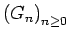given by the recurrence Gn = Gn-1 + Gn-2 for all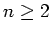with integral initial conditions G0 and G1. In particular, we give precise values for the greatest common divisor (GCD) of all sums of k consecutive terms of. When G0 = 0 and G1 = 1, we yield the GCD of all sums of k consecutive Fibonacci numbers, and when G0 = 2 and G1 = 1, we yield the GCD of all sums of k consecutive Lucas numbers. Denoting the GCD of all sums of k consecutive generalized Fibonacci numbers by the symbol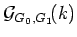, we give two tantalizing characterizations for these values, one involving a simple formula in k and another involving generalized Pisano periods: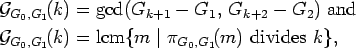where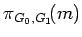denotes the generalized Pisano period of the generalized Fibonacci sequence modulo m. The fact that these vastly different-looking formulas coincide leads to some surprising and delightful new understandings of the Fibonacci and Lucas numbers.

Full version:  pdf,    dvi,    ps,    latex

(Concerned with sequences A210209 A229339.)

Received May 25 2021; revised version received November 3 2021. Published in Journal of Integer Sequences, November 3 2021.